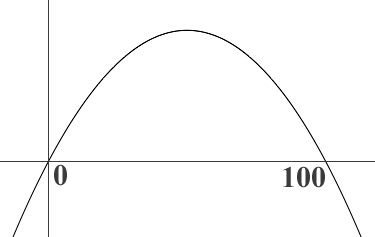SEARCH HOMEMath Central Quandaries & QueriesQuestion from Adiba, a student: A bridge constructed over a bayou has a supporting arch in the shape of a parabola .Find the equation of the parabolic arch if the length of the road over the arch is 100 meters and the maximum height of the arch is 40 meters. I did the problem but not sure is it correct . I did like this f(0)=0 we get c=40 if we took quadratic equation in x for a down ward parabola then how to find b and a please show me the answer of either a or b .Or I should use the standard form of parabola y=a(x-h)^2+k then how to find a,h,k please help meDid you draw a diagram?If the parabola is $y = f(x)$ then you are correct that with the axes as in my diagram $f(0) = 0.$ but also $f(100) = 0$ so $0$ and $100$ are roots of $f(x).$ Since it is a parabola (quadratic) these are the only roots so $f(x) = k x(x - 100)$ for some constant $k.$

The maximum height of 40 meters occurs when $x = 50$ meters. What is $k?$

PennyMath Central is supported by the University of Regina and The Pacific Institute for the Mathematical Sciences.